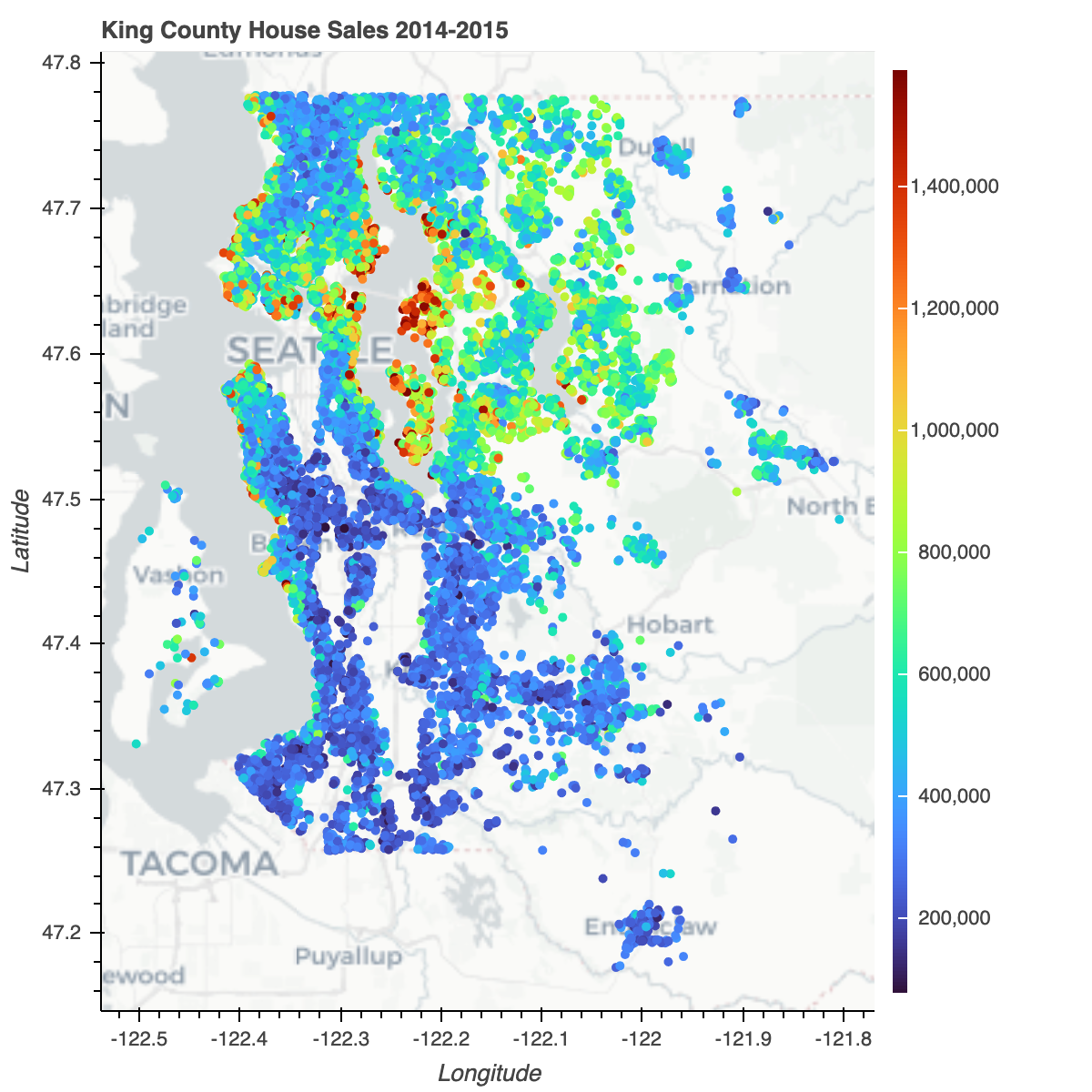1648895520

## Bokeh: Interactive Data Visualization In The Browser From Python

Bokeh is an interactive visualization library for modern web browsers. It provides elegant, concise construction of versatile graphics, and affords high-performance interactivity over large or streaming datasets. Bokeh can help anyone who would like to quickly and easily make interactive plots, dashboards, and data applications.

If you like Bokeh and would like to support our mission, please consider making a donation.

## Installation

The easiest way to install Bokeh is using the Anaconda Python distribution and its included Conda package management system. To install Bokeh and its required dependencies, enter the following command at a Bash or Windows command prompt:

conda install bokeh


To install using pip, enter the following command at a Bash or Windows command prompt:

pip install bokeh


## Resources

Once Bokeh is installed, check out the first steps guides.

Visit the full documentation site to view the User's Guide or launch the Bokeh tutorial to learn about Bokeh in live Jupyter Notebooks.

Community support is available on the Project Discourse.

If you would like to contribute to Bokeh, please review the Contributor Guide and request an invitation to the Bokeh Dev Slack workspace.

Note: Everyone interacting in the Bokeh project's codebases, issue trackers and discussion forums is expected to follow the Code of Conduct.

## Support

### Fiscal Support

The Bokeh project is grateful for individual contributions sponsorship as well as support by the organizations and companies below:

Bokeh is a Sponsored Project of NumFOCUS, a 501(c)(3) nonprofit charity in the United States. NumFOCUS provides Bokeh with fiscal, legal, and administrative support to help ensure the health and sustainability of the project. Visit numfocus.org for more information.

Donations to Bokeh are managed by NumFOCUS. For donors in the United States, your gift is tax-deductible to the extent provided by law. As with any donation, you should consult with your tax adviser about your particular tax situation.

### In-kind Support

The Bokeh project is also grateful for the donation of services from the following companies:

Author: bokeh
Source Code: https://github.com/bokeh/bokeh1642995900

## Pandas Bokeh: Bokeh Plotting Backend for Pandas and GeoPandas

Pandas-Bokeh provides a Bokeh plotting backend for Pandas, GeoPandas and Pyspark DataFrames, similar to the already existing Visualization feature of Pandas. Importing the library adds a complementary plotting method plot_bokeh() on DataFrames and Series.

With Pandas-Bokeh, creating stunning, interactive, HTML-based visualization is as easy as calling:

df.plot_bokeh()


Pandas-Bokeh also provides native support as a Pandas Plotting backend for Pandas >= 0.25. When Pandas-Bokeh is installed, switchting the default Pandas plotting backend to Bokeh can be done via:

pd.set_option('plotting.backend', 'pandas_bokeh')


More details about the new Pandas backend can be found below.

## Interactive Documentation

https://patrikhlobil.github.io/Pandas-Bokeh/

for an interactive version of the documentation below, where you can play with the dynamic Bokeh plots.

For more information have a look at the Examples below or at notebooks on the Github Repository of this project.## Installation

You can install Pandas-Bokeh from PyPI via pip

pip install pandas-bokeh


or conda:

conda install -c patrikhlobil pandas-bokeh


With the current release 0.5.5, Pandas-Bokeh officially supports Python 3.6 and newer. For more details, see Release Notes.

## How To Use

### Classical Use

The Pandas-Bokeh library should be imported after Pandas, GeoPandas and/or Pyspark. After the import, one should define the plotting output, which can be:

• pandas_bokeh.output_notebook(): Embeds the Plots in the cell outputs of the notebook. Ideal when working in Jupyter Notebooks.
• pandas_bokeh.output_file(filename): Exports the plot to the provided filename as an HTML.

For more details about the plotting outputs, see the reference here or the Bokeh documentation.

import pandas as pd
import pandas_bokeh
pandas_bokeh.output_notebook()


import pandas as pd
import pandas_bokeh
pandas_bokeh.output_file("Interactive Plot.html")


### Pandas-Bokeh as native Pandas plotting backend

For pandas >= 0.25, a plotting backend switch is natively supported. It can be achievied by calling:

import pandas as pd
pd.set_option('plotting.backend', 'pandas_bokeh')


Now, the plotting API is accessible for a Pandas DataFrame via:

df.plot(...)


All additional functionalities of Pandas-Bokeh are then accessible at pd.plotting. So, setting the output to notebook is:

pd.plotting.output_notebook()


or calling the grid layout functionality:

pd.plotting.plot_grid(...)


Note: Backwards compatibility is kept since there will still be the df.plot_bokeh(...) methods for a DataFrame.

### Plot types

Supported plottypes are at the moment:

Also, check out the complementary chapter Outputs, Formatting & Layouts about:

## Lineplot

### Basic Lineplot

This simple lineplot in Pandas-Bokeh already contains various interactive elements:

• a pannable and zoomable (zoom in plotarea and zoom on axis) plot
• by clicking on the legend elements, one can hide and show the individual lines
• a Hovertool for the plotted lines

Consider the following simple example:

import numpy as np

np.random.seed(42)
"Apple": np.random.randn(1000)+0.17},
index=pd.date_range('1/1/2000', periods=1000))
df = df.cumsum()
df = df + 50
df.plot_bokeh(kind="line")       #equivalent to df.plot_bokeh.line()Note, that similar to the regular pandas.DataFrame.plot method, there are also additional accessors to directly access the different plotting types like:

• df.plot_bokeh(kind="line", ...)df.plot_bokeh.line(...)
• df.plot_bokeh(kind="bar", ...)df.plot_bokeh.bar(...)
• df.plot_bokeh(kind="hist", ...)df.plot_bokeh.hist(...)
• ...

There are various optional parameters to tune the plots, for example:

• kind: Which kind of plot should be produced. Currently supported are: "line", "point", "scatter", "bar" and "histogram". In the near future many more will be implemented as horizontal barplot, boxplots, pie-charts, etc.
• x: Name of the column to use for the horizontal x-axis. If the x parameter is not specified, the index is used for the x-values of the plot. Alternative, also an array of values can be passed that has the same number of elements as the DataFrame.
• y: Name of column or list of names of columns to use for the vertical y-axis.
• figsize: Choose width & height of the plot
• title: Sets title of the plot
• xlim/ylim: Set visibler range of plot for x- and y-axis (also works for datetime x-axis)
• xlabel/ylabel: Set x- and y-labels
• logx/logy: Set log-scale on x-/y-axis
• xticks/yticks: Explicitly set the ticks on the axes
• color: Defines a single color for a plot.
• colormap: Can be used to specify multiple colors to plot. Can be either a list of colors or the name of a Bokeh color palette
• hovertool: If True a Hovertool is active, else if False no Hovertool is drawn.
• hovertool_string: If specified, this string will be used for the hovertool (@{column} will be replaced by the value of the column for the element the mouse hovers over, see also Bokeh documentation and here)
• toolbar_location: Specify the position of the toolbar location (None, "above", "below", "left" or "right"). Default: "right"
• zooming: Enables/Disables zooming. Default: True
• panning: Enables/Disables panning. Default: True
• fontsize_label/fontsize_ticks/fontsize_title/fontsize_legend: Set fontsize of labels, ticks, title or legend (int or string of form "15pt")
• rangetool Enables a range tool scroller. Default False
• kwargs**: Optional keyword arguments of bokeh.plotting.figure.line

Try them out to get a feeling for the effects. Let us consider now:

df.plot_bokeh.line(
figsize=(800, 450),
y="Apple",
xlabel="Date",
ylabel="Stock price [$]", yticks=[0, 100, 200, 300, 400], ylim=(0, 400), toolbar_location=None, colormap=["red", "blue"], hovertool_string=r"""<img src='https://upload.wikimedia.org/wikipedia/commons/thumb/f/fa/Apple_logo_black.svg/170px-Apple_logo_black.svg.png' height="42" alt="@imgs" width="42" style="float: left; margin: 0px 15px 15px 0px;" border="2"></img> Apple <h4> Stock Price: </h4> @{Apple}""", panning=False, zooming=False)#### Lineplot with data points For lineplots, as for many other plot-kinds, there are some special keyword arguments that only work for this plotting type. For lineplots, these are: • plot_data_points: Plot also the data points on the lines • plot_data_points_size: Determines the size of the data points • marker: Defines the point type (Default: "circle"). Possible values are: 'circle', 'square', 'triangle', 'asterisk', 'circle_x', 'square_x', 'inverted_triangle', 'x', 'circle_cross', 'square_cross', 'diamond', 'cross' • kwargs**: Optional keyword arguments of bokeh.plotting.figure.line Let us use this information to have another version of the same plot: df.plot_bokeh.line( figsize=(800, 450), title="Apple vs Google", xlabel="Date", ylabel="Stock price [$]",
yticks=[0, 100, 200, 300, 400],
ylim=(100, 200),
xlim=("2001-01-01", "2001-02-01"),
colormap=["red", "blue"],
plot_data_points=True,
plot_data_points_size=10,
marker="asterisk")#### Lineplot with rangetool

ts = pd.Series(np.random.randn(1000), index=pd.date_range('1/1/2000', periods=1000))
df = pd.DataFrame(np.random.randn(1000, 4), index=ts.index, columns=list('ABCD'))
df = df.cumsum()

df.plot_bokeh(rangetool=True)## Pointplot

If you just wish to draw the date points for curves, the pointplot option is the right choice. It also accepts the kwargs of bokeh.plotting.figure.scatter like marker or size:

import numpy as np

x = np.arange(-3, 3, 0.1)
y2 = x**2
y3 = x**3
df = pd.DataFrame({"x": x, "Parabula": y2, "Cube": y3})
df.plot_bokeh.point(
x="x",
xticks=range(-3, 4),
size=5,
colormap=["#009933", "#ff3399"],
title="Pointplot (Parabula vs. Cube)",
marker="x")## Stepplot

With a similar API as the line- & pointplots, one can generate a stepplot. Additional keyword arguments for this plot type are passes to bokeh.plotting.figure.step, e.g. mode (before, after, center), see the following example

import numpy as np

x = np.arange(-3, 3, 1)
y2 = x**2
y3 = x**3
df = pd.DataFrame({"x": x, "Parabula": y2, "Cube": y3})
df.plot_bokeh.step(
x="x",
xticks=range(-1, 1),
colormap=["#009933", "#ff3399"],
title="Pointplot (Parabula vs. Cube)",
figsize=(800,300),
fontsize_title=30,
fontsize_label=25,
fontsize_ticks=15,
fontsize_legend=5,
)

df.plot_bokeh.step(
x="x",
xticks=range(-1, 1),
colormap=["#009933", "#ff3399"],
title="Pointplot (Parabula vs. Cube)",
mode="after",
figsize=(800,300)
)Note that the step-plot API of Bokeh does so far not support a hovertool functionality.

## Scatterplot

A basic scatterplot can be created using the kind="scatter" option. For scatterplots, the x and y parameters have to be specified and the following optional keyword argument is allowed:

category: Determines the category column to use for coloring the scatter points

kwargs**: Optional keyword arguments of bokeh.plotting.figure.scatter

Note, that the pandas.DataFrame.plot_bokeh() method return per default a Bokeh figure, which can be embedded in Dashboard layouts with other figures and Bokeh objects (for more details about (sub)plot layouts and embedding the resulting Bokeh plots as HTML click here).

In the example below, we use the building grid layout support of Pandas-Bokeh to display both the DataFrame (using a Bokeh DataTable) and the resulting scatterplot:

# Load Iris Dataset:
r"https://raw.githubusercontent.com/PatrikHlobil/Pandas-Bokeh/master/docs/Testdata/iris/iris.csv"
)
df = df.sample(frac=1)

# Create Bokeh-Table with DataFrame:
from bokeh.models.widgets import DataTable, TableColumn
from bokeh.models import ColumnDataSource

data_table = DataTable(
columns=[TableColumn(field=Ci, title=Ci) for Ci in df.columns],
source=ColumnDataSource(df),
height=300,
)

# Create Scatterplot:
p_scatter = df.plot_bokeh.scatter(
x="petal length (cm)",
y="sepal width (cm)",
category="species",
title="Iris DataSet Visualization",
show_figure=False,
)

# Combine Table and Scatterplot via grid layout:
pandas_bokeh.plot_grid([[data_table, p_scatter]], plot_width=400, plot_height=350)A possible optional keyword parameters that can be passed to bokeh.plotting.figure.scatter is size. Below, we use the sepal length of the Iris data as reference for the size:

#Change one value to clearly see the effect of the size keyword
df.loc[13, "sepal length (cm)"] = 15

#Make scatterplot:
p_scatter = df.plot_bokeh.scatter(
x="petal length (cm)",
y="sepal width (cm)",
category="species",
title="Iris DataSet Visualization with Size Keyword",
size="sepal length (cm)")In this example you can see, that the additional dimension sepal length cannot be used to clearly differentiate between the virginica and versicolor species.

## Barplot

The barplot API has no special keyword arguments, but accepts optional kwargs of bokeh.plotting.figure.vbar like alpha. It uses per default the index for the bar categories (however, also columns can be used as x-axis category using the x argument).

data = {
'fruits':
['Apples', 'Pears', 'Nectarines', 'Plums', 'Grapes', 'Strawberries'],
'2015': [2, 1, 4, 3, 2, 4],
'2016': [5, 3, 3, 2, 4, 6],
'2017': [3, 2, 4, 4, 5, 3]
}
df = pd.DataFrame(data).set_index("fruits")

p_bar = df.plot_bokeh.bar(
ylabel="Price per Unit [€]",
title="Fruit prices per Year",
alpha=0.6)Using the stacked keyword argument you also maked stacked barplots:

p_stacked_bar = df.plot_bokeh.bar(
ylabel="Price per Unit [€]",
title="Fruit prices per Year",
stacked=True,
alpha=0.6)Also horizontal versions of the above barplot are supported with the keyword kind="barh" or the accessor plot_bokeh.barh. You can still specify a column of the DataFrame as the bar category via the x argument if you do not wish to use the index.

#Reset index, such that "fruits" is now a column of the DataFrame:
df.reset_index(inplace=True)

#Create horizontal bar (via kind keyword):
p_hbar = df.plot_bokeh(
kind="barh",
x="fruits",
xlabel="Price per Unit [€]",
title="Fruit prices per Year",
alpha=0.6,
legend = "bottom_right",
show_figure=False)

#Create stacked horizontal bar (via barh accessor):
p_stacked_hbar = df.plot_bokeh.barh(
x="fruits",
stacked=True,
xlabel="Price per Unit [€]",
title="Fruit prices per Year",
alpha=0.6,
legend = "bottom_right",
show_figure=False)

#Plot all barplot examples in a grid:
pandas_bokeh.plot_grid([[p_bar, p_stacked_bar],
[p_hbar, p_stacked_hbar]],
plot_width=450)## Histogram

For drawing histograms (kind="hist"), Pandas-Bokeh has a lot of customization features. Optional keyword arguments for histogram plots are:

• bins: Determines bins to use for the histogram. If bins is an int, it defines the number of equal-width bins in the given range (10, by default). If bins is a sequence, it defines the bin edges, including the rightmost edge, allowing for non-uniform bin widths. If bins is a string, it defines the method used to calculate the optimal bin width, as defined by histogram_bin_edges.
• histogram_type: Either "sidebyside", "topontop" or "stacked". Default: "topontop"
• stacked: Boolean that overrides the histogram_type as "stacked" if given. Default: False
• kwargs**: Optional keyword arguments of bokeh.plotting.figure.quad

Below examples of the different histogram types:

import numpy as np

df_hist = pd.DataFrame({
'a': np.random.randn(1000) + 1,
'b': np.random.randn(1000),
'c': np.random.randn(1000) - 1
},
columns=['a', 'b', 'c'])

#Top-on-Top Histogram (Default):
df_hist.plot_bokeh.hist(
bins=np.linspace(-5, 5, 41),
vertical_xlabel=True,
hovertool=False,
title="Normal distributions (Top-on-Top)",
line_color="black")

#Side-by-Side Histogram (multiple bars share bin side-by-side) also accessible via
#kind="hist":
df_hist.plot_bokeh(
kind="hist",
bins=np.linspace(-5, 5, 41),
histogram_type="sidebyside",
vertical_xlabel=True,
hovertool=False,
title="Normal distributions (Side-by-Side)",
line_color="black")

#Stacked histogram:
df_hist.plot_bokeh.hist(
bins=np.linspace(-5, 5, 41),
histogram_type="stacked",
vertical_xlabel=True,
hovertool=False,
title="Normal distributions (Stacked)",
line_color="black")Further, advanced keyword arguments for histograms are:

• weights: A column of the DataFrame that is used as weight for the histogramm aggregation (see also numpy.histogram)
• normed: If True, histogram values are normed to 1 (sum of histogram values=1). It is also possible to pass an integer, e.g. normed=100 would result in a histogram with percentage y-axis (sum of histogram values=100). Default: False
• cumulative: If True, a cumulative histogram is shown. Default: False
• show_average: If True, the average of the histogram is also shown. Default: False

Their usage is shown in these examples:

p_hist = df_hist.plot_bokeh.hist(
y=["a", "b"],
bins=np.arange(-4, 6.5, 0.5),
normed=100,
vertical_xlabel=True,
ylabel="Share[%]",
title="Normal distributions (normed)",
show_average=True,
xlim=(-4, 6),
ylim=(0, 30),
show_figure=False)

p_hist_cum = df_hist.plot_bokeh.hist(
y=["a", "b"],
bins=np.arange(-4, 6.5, 0.5),
normed=100,
cumulative=True,
vertical_xlabel=True,
ylabel="Share[%]",
title="Normal distributions (normed & cumulative)",
show_figure=False)

pandas_bokeh.plot_grid([[p_hist, p_hist_cum]], plot_width=450, plot_height=300)## Areaplot

Areaplot (kind="area") can be either drawn on top of each other or stacked. The important parameters are:

stacked: If True, the areaplots are stacked. If False, plots are drawn on top of each other. Default: False

kwargs**: Optional keyword arguments of bokeh.plotting.figure.patch

Let us consider the energy consumption split by source that can be downloaded as DataFrame via:

df_energy = pd.read_csv(r"https://raw.githubusercontent.com/PatrikHlobil/Pandas-Bokeh/master/docs/Testdata/energy/energy.csv",
parse_dates=["Year"])


Creating the Areaplot can be achieved via:

df_energy.plot_bokeh.area(
x="Year",
stacked=True,
legend="top_left",
colormap=["brown", "orange", "black", "grey", "blue", "green"],
title="Worldwide energy consumption split by energy source",
ylabel="Million tonnes oil equivalent",
ylim=(0, 16000))Note that the energy consumption of fossile energy is still increasing and renewable energy sources are still small in comparison 😢!!! However, when we norm the plot using the normed keyword, there is a clear trend towards renewable energies in the last decade:

df_energy.plot_bokeh.area(
x="Year",
stacked=True,
normed=100,
legend="bottom_left",
colormap=["brown", "orange", "black", "grey", "blue", "green"],
title="Worldwide energy consumption split by energy source",
ylabel="Million tonnes oil equivalent")## Pieplot

For Pieplots, let us consider a dataset showing the results of all Bundestags elections in Germany since 2002:

df_pie = pd.read_csv(r"https://raw.githubusercontent.com/PatrikHlobil/Pandas-Bokeh/master/docs/Testdata/Bundestagswahl/Bundestagswahl.csv")
df_pie


We can create a Pieplot of the last election in 2017 by specifying the "Partei" (german for party) column as the x column and the "2017" column as the y column for values:

df_pie.plot_bokeh.pie(
x="Partei",
y="2017",
colormap=["blue", "red", "yellow", "green", "purple", "orange", "grey"],
title="Results of German Bundestag Election 2017",
)When you pass several columns to the y parameter (not providing the y-parameter assumes you plot all columns), multiple nested pieplots will be shown in one plot:

df_pie.plot_bokeh.pie(
x="Partei",
colormap=["blue", "red", "yellow", "green", "purple", "orange", "grey"],
title="Results of German Bundestag Elections [2002-2017]",
line_color="grey")## Mapplot

The mapplot method of Pandas-Bokeh allows for plotting geographic points stored in a Pandas DataFrame on an interactive map. For more advanced Geoplots for line and polygon shapes have a look at the Geoplots examples for the GeoPandas API of Pandas-Bokeh.

For mapplots, only (latitude, longitude) pairs in geographic projection (WGS84) can be plotted on a map. The basic API has the following 2 base parameters:

• x: name of the longitude column of the DataFrame
• y: name of the latitude column of the DataFrame

The other optional keyword arguments are discussed in the section about the GeoPandas API, e.g. category for coloring the points.

Below an example of plotting all cities for more than 1 million inhabitants:

df_mapplot = pd.read_csv(r"https://raw.githubusercontent.com/PatrikHlobil/Pandas-Bokeh/master/docs/Testdata/populated%20places/populated_places.csv")

df_mapplot["size"] = df_mapplot["pop_max"] / 1000000
df_mapplot.plot_bokeh.map(
x="longitude",
y="latitude",
hovertool_string="""<h2> @{name} </h2>

<h3> Population: @{pop_max} </h3>""",
tile_provider="STAMEN_TERRAIN_RETINA",
size="size",
figsize=(900, 600),
title="World cities with more than 1.000.000 inhabitants")## Geoplots

Pandas-Bokeh also allows for interactive plotting of Maps using GeoPandas by providing a geopandas.GeoDataFrame.plot_bokeh() method. It allows to plot the following geodata on a map :

• Points/MultiPoints
• Lines/MultiLines
• Polygons/MultiPolygons

Note: t is not possible to mix up the objects types, i.e. a GeoDataFrame with Points and Lines is for example not allowed.

Les us start with a simple example using the "World Borders Dataset" . Let us first import all neccessary libraries and read the shapefile:

import geopandas as gpd
import pandas as pd
import pandas_bokeh
pandas_bokeh.output_notebook()



Plotting the data on a map is as simple as calling:

df_states.plot_bokeh(simplify_shapes=10000)We also passed the optional parameter simplify_shapes (~meter) to improve plotting performance (for a reference see shapely.object.simplify). The above geolayer thus has an accuracy of about 10km.

Many keyword arguments like xlabel, ylabel, xlim, ylim, title, colormap, hovertool, zooming, panning, ... for costumizing the plot are also available for the geoplotting API and can be uses as in the examples shown above. There are however also many other options especially for plotting geodata:

• geometry_column: Specify the column that stores the geometry-information (default: "geometry")
• hovertool_columns: Specify column names, for which values should be shown in hovertool
• hovertool_string: If specified, this string will be used for the hovertool (@{column} will be replaced by the value of the column for the element the mouse hovers over, see also Bokeh documentation)
• colormap_uselog: If set True, the colormapper is using a logscale. Default: False
• colormap_range: Specify the value range of the colormapper via (min, max) tuple
• tile_provider: Define build-in tile provider for background maps. Possible values: None, 'CARTODBPOSITRON', 'CARTODBPOSITRON_RETINA', 'STAMEN_TERRAIN', 'STAMEN_TERRAIN_RETINA', 'STAMEN_TONER', 'STAMEN_TONER_BACKGROUND', 'STAMEN_TONER_LABELS'. Default: CARTODBPOSITRON_RETINA
• tile_provider_url: An arbitraty tile_provider_url of the form '/{Z}/{X}/{Y}*.png' can be passed to be used as background map.
• tile_attribution: String (also HTML accepted) for showing attribution for tile source in the lower right corner
• tile_alpha: Sets the alpha value of the background tile between [0, 1]. Default: 1

One of the most common usage of map plots are choropleth maps, where the color of a the objects is determined by the property of the object itself. There are 3 ways of drawing choropleth maps using Pandas-Bokeh, which are described below.

### Categories

This is the simplest way. Just provide the category keyword for the selection of the property column:

• category: Specifies the column of the GeoDataFrame that should be used to draw a choropleth map
• show_colorbar: Whether or not to show a colorbar for categorical plots. Default: True

Let us now draw the regions as a choropleth plot using the category keyword (at the moment, only numerical columns are supported for choropleth plots):

df_states.plot_bokeh(
figsize=(900, 600),
simplify_shapes=5000,
category="REGION",
show_colorbar=False,
colormap=["blue", "yellow", "green", "red"],
hovertool_columns=["STATE_NAME", "REGION"],
tile_provider="STAMEN_TERRAIN_RETINA")


When hovering over the states, the state-name and the region are shown as specified in the hovertool_columns argument.### Dropdown

By passing a list of column names of the GeoDataFrame as the dropdown keyword argument, a dropdown menu is shown above the map. This dropdown menu can be used to select the choropleth layer by the user. :

df_states["STATE_NAME_SMALL"] = df_states["STATE_NAME"].str.lower()

df_states.plot_bokeh(
figsize=(900, 600),
simplify_shapes=5000,
dropdown=["POPESTIMATE2010", "POPESTIMATE2017"],
colormap="Viridis",
hovertool_string="""
<img
src="https://www.states101.com/img/flags/gif/small/@STATE_NAME_SMALL.gif"
height="42" alt="@imgs" width="42"
style="float: left; margin: 0px 15px 15px 0px;"
border="2"></img>

<h2>  @STATE_NAME </h2>
<h3> 2010: @POPESTIMATE2010 </h3>
<h3> 2017: @POPESTIMATE2017 </h3>""",
tile_provider_url=r"http://c.tile.stamen.com/watercolor/{Z}/{X}/{Y}.jpg",
tile_attribution='Map tiles by <a href="http://stamen.com">Stamen Design</a>, under <a href="http://creativecommons.org/licenses/by/3.0">CC BY 3.0</a>. Data by <a href="http://openstreetmap.org">OpenStreetMap</a>, under <a href="http://www.openstreetmap.org/copyright">ODbL</a>.'
)Using hovertool_string, one can pass a string that can contain arbitrary HTML elements (including divs, images, ...) that is shown when hovering over the geographies (@{column} will be replaced by the value of the column for the element the mouse hovers over, see also Bokeh documentation).

Here, we also used an OSM tile server with watercolor style via tile_provider_url and added the attribution via tile_attribution.

### Sliders

Another option for interactive choropleth maps is the slider implementation of Pandas-Bokeh. The possible keyword arguments are here:

• slider: By passing a list of column names of the GeoDataFrame, a slider can be used to . This dropdown menu can be used to select the choropleth layer by the user.
• slider_range: Pass a range (or numpy.arange) of numbers object to relate the sliders values with the slider columns. By passing range(0,10), the slider will have values [0, 1, 2, ..., 9], when passing numpy.arange(3,5,0.5), the slider will have values [3, 3.5, 4, 4.5]. Default: range(0, len(slider))
• slider_name: Specifies the title of the slider. Default is an empty string.

This can be used to display the change in population relative to the year 2010:

#Calculate change of population relative to 2010:
for i in range(8):
df_states["Delta_Population_201%d"%i] = ((df_states["POPESTIMATE201%d"%i] / df_states["POPESTIMATE2010"]) -1 ) * 100

#Specify slider columns:
slider_columns = ["Delta_Population_201%d"%i for i in range(8)]

#Specify slider-range (Maps "Delta_Population_2010" -> 2010,
#                           "Delta_Population_2011" -> 2011, ...):
slider_range = range(2010, 2018)

#Make slider plot:
df_states.plot_bokeh(
figsize=(900, 600),
simplify_shapes=5000,
slider=slider_columns,
slider_range=slider_range,
slider_name="Year",
colormap="Inferno",
hovertool_columns=["STATE_NAME"] + slider_columns,
title="Change of Population [%]")### Plot multiple geolayers

If you wish to display multiple geolayers, you can pass the Bokeh figure of a Pandas-Bokeh plot via the figure keyword to the next plot_bokeh() call:

import geopandas as gpd
import pandas_bokeh
pandas_bokeh.output_notebook()

# Read in GeoJSONs from URL:
r"https://raw.githubusercontent.com/PatrikHlobil/Pandas-Bokeh/master/docs/Testdata/populated%20places/ne_10m_populated_places_simple_bigcities.geojson"
)
df_cities["size"] = df_cities.pop_max / 400000

#Plot shapes of US states (pass figure options to this initial plot):
figure = df_states.plot_bokeh(
figsize=(800, 450),
simplify_shapes=10000,
show_figure=False,
xlim=[-170, -80],
ylim=[10, 70],
category="REGION",
colormap="Dark2",
legend="States",
show_colorbar=False,
)

#Plot cities as points on top of the US states layer by passing the figure:
df_cities.plot_bokeh(
figure=figure,         # <== pass figure here!
category="pop_max",
colormap="Viridis",
colormap_uselog=True,
size="size",
hovertool_string="""<h1>@name</h1>
<h3>Population: @pop_max </h3>""",
marker="inverted_triangle",
legend="Cities",
)### Point & Line plots:

Below, you can see an example that use Pandas-Bokeh to plot point data on a map. The plot shows all cities with a population larger than 1.000.000. For point plots, you can select the marker as keyword argument (since it is passed to bokeh.plotting.figure.scatter). Here an overview of all available marker types:

gdf = gpd.read_file(r"https://raw.githubusercontent.com/PatrikHlobil/Pandas-Bokeh/master/docs/Testdata/populated%20places/ne_10m_populated_places_simple_bigcities.geojson")
gdf["size"] = gdf.pop_max / 400000

gdf.plot_bokeh(
category="pop_max",
colormap="Viridis",
colormap_uselog=True,
size="size",
hovertool_string="""<h1>@name</h1>
<h3>Population: @pop_max </h3>""",
xlim=[-15, 35],
ylim=[30,60],
marker="inverted_triangle");In a similar way, also GeoDataFrames with (multi)line shapes can be drawn using Pandas-Bokeh.

### Colorbar formatting:

If you want to display the numerical labels on your colorbar with an alternative to the scientific format, you can pass in a one of the bokeh number string formats or an instance of one of the bokeh.models.formatters to the colorbar_tick_format argument in the geoplot

An example of using the string format argument:

df_states = gpd.read_file(r"https://raw.githubusercontent.com/PatrikHlobil/Pandas-Bokeh/master/docs/Testdata/states/states.geojson")

df_states["STATE_NAME_SMALL"] = df_states["STATE_NAME"].str.lower()

# pass in a string format to colorbar_tick_format to display the ticks as 10m rather than 1e7
df_states.plot_bokeh(
figsize=(900, 600),
category="POPESTIMATE2017",
simplify_shapes=5000,
colormap="Inferno",
colormap_uselog=True,
colorbar_tick_format="0.0a")An example of using the bokeh PrintfTickFormatter:

df_states = gpd.read_file(r"https://raw.githubusercontent.com/PatrikHlobil/Pandas-Bokeh/master/docs/Testdata/states/states.geojson")

df_states["STATE_NAME_SMALL"] = df_states["STATE_NAME"].str.lower()

for i in range(8):
df_states["Delta_Population_201%d"%i] = ((df_states["POPESTIMATE201%d"%i] / df_states["POPESTIMATE2010"]) -1 ) * 100

# pass in a PrintfTickFormatter instance colorbar_tick_format to display the ticks with 2 decimal places
df_states.plot_bokeh(
figsize=(900, 600),
category="Delta_Population_2017",
simplify_shapes=5000,
colormap="Inferno",
colorbar_tick_format=PrintfTickFormatter(format="%4.2f"))## Outputs, Formatting & Layouts

### Output options

The pandas.DataFrame.plot_bokeh API has the following additional keyword arguments:

• show_figure: If True, the resulting figure is shown (either in the notebook or exported and shown as HTML file, see Basics. If False, None is returned. Default: True
• return_html: If True, the method call returns an HTML string that contains all Bokeh CSS&JS resources and the figure embedded in a div. This HTML representation of the plot can be used for embedding the plot in an HTML document. Default: False

If you have a Bokeh figure or layout, you can also use the pandas_bokeh.embedded_html function to generate an embeddable HTML representation of the plot. This can be included into any valid HTML (note that this is not possible directly with the HTML generated by the pandas_bokeh.output_file output option, because it includes an HTML header). Let us consider the following simple example:

#Import Pandas and Pandas-Bokeh (if you do not specify an output option, the standard is
#output_file):
import pandas as pd
import pandas_bokeh

#Create DataFrame to Plot:
import numpy as np
x = np.arange(-10, 10, 0.1)
sin = np.sin(x)
cos = np.cos(x)
tan = np.tan(x)
df = pd.DataFrame({"x": x, "sin(x)": sin, "cos(x)": cos, "tan(x)": tan})

#Make Bokeh plot from DataFrame using Pandas-Bokeh. Do not show the plot, but export
#it to an embeddable HTML string:
html_plot = df.plot_bokeh(
kind="line",
x="x",
y=["sin(x)", "cos(x)", "tan(x)"],
xticks=range(-20, 20),
title="Trigonometric functions",
show_figure=False,
return_html=True,
ylim=(-1.5, 1.5))

#Write some HTML and embed the HTML plot below it. For production use, please use
#Templates and the awesome Jinja library.
html = r"""
<script type="text/x-mathjax-config">
MathJax.Hub.Config({tex2jax: {inlineMath: [['$','$'], ['\$','\$']]}});
</script>
<script type="text/javascript"
src="http://cdn.mathjax.org/mathjax/latest/MathJax.js?config=TeX-AMS-MML_HTMLorMML">
</script>

<h1> Trigonometric functions </h1>

<p> The basic trigonometric functions are:</p>

<p>$sin(x)$</p>
<p>$cos(x)$</p>
<p>$tan(x) = \frac{sin(x)}{cos(x)}$</p>

<p>Below is a plot that shows them</p>

""" + html_plot

#Export the HTML string to an external HTML file and show it:
with open("test.html" , "w") as f:
f.write(html)

import webbrowser
webbrowser.open("test.html")


This code will open up a webbrowser and show the following page. As you can see, the interactive Bokeh plot is embedded nicely into the HTML layout. The return_html option is ideal for the use in a templating engine like Jinja.### Auto Scaling Plots

For single plots that have a number of x axis values or for larger monitors, you can auto scale the figure to the width of the entire jupyter cell by setting the sizing_mode parameter.

df = pd.DataFrame(np.random.rand(10, 4), columns=['a', 'b', 'c', 'd'])

df.plot_bokeh(kind="bar", figsize=(500, 200), sizing_mode="scale_width")The figsize parameter can be used to change the height and width as well as act as a scaling multiplier against the axis that is not being scaled.

### Number formats

To change the formats of numbers in the hovertool, use the number_format keyword argument. For a documentation about the format to pass, have a look at the Bokeh documentation.Let us consider some examples for the number 3.141592653589793:

This number format will be applied to all numeric columns of the hovertool. If you want to make a very custom or complicated hovertool, you should probably use the hovertool_string keyword argument, see e.g. this example. Below, we use the number_format parameter to specify the "Stock Price" format to 2 decimal digits and an additional $sign. import numpy as np #Lineplot: np.random.seed(42) df = pd.DataFrame({ "Google": np.random.randn(1000) + 0.2, "Apple": np.random.randn(1000) + 0.17 }, index=pd.date_range('1/1/2000', periods=1000)) df = df.cumsum() df = df + 50 df.plot_bokeh( kind="line", title="Apple vs Google", xlabel="Date", ylabel="Stock price [$]",
yticks=[0, 100, 200, 300, 400],
ylim=(0, 400),
colormap=["red", "blue"],
number_format="1.00 $")#### Suppress scientific notation for axes If you want to suppress the scientific notation for axes, you can use the disable_scientific_axes parameter, which accepts one of "x", "y", "xy": df = pd.DataFrame({"Animal": ["Mouse", "Rabbit", "Dog", "Tiger", "Elefant", "Wale"], "Weight [g]": [19, 3000, 40000, 200000, 6000000, 50000000]}) p_scientific = df.plot_bokeh(x="Animal", y="Weight [g]", show_figure=False) p_non_scientific = df.plot_bokeh(x="Animal", y="Weight [g]", disable_scientific_axes="y", show_figure=False,) pandas_bokeh.plot_grid([[p_scientific, p_non_scientific]], plot_width = 450)### Dashboard Layouts As shown in the Scatterplot Example, combining plots with plots or other HTML elements is straighforward in Pandas-Bokeh due to the layout capabilities of Bokeh. The easiest way to generate a dashboard layout is using the pandas_bokeh.plot_grid method (which is an extension of bokeh.layouts.gridplot): import pandas as pd import numpy as np import pandas_bokeh pandas_bokeh.output_notebook() #Barplot: data = { 'fruits': ['Apples', 'Pears', 'Nectarines', 'Plums', 'Grapes', 'Strawberries'], '2015': [2, 1, 4, 3, 2, 4], '2016': [5, 3, 3, 2, 4, 6], '2017': [3, 2, 4, 4, 5, 3] } df = pd.DataFrame(data).set_index("fruits") p_bar = df.plot_bokeh( kind="bar", ylabel="Price per Unit [€]", title="Fruit prices per Year", show_figure=False) #Lineplot: np.random.seed(42) df = pd.DataFrame({ "Google": np.random.randn(1000) + 0.2, "Apple": np.random.randn(1000) + 0.17 }, index=pd.date_range('1/1/2000', periods=1000)) df = df.cumsum() df = df + 50 p_line = df.plot_bokeh( kind="line", title="Apple vs Google", xlabel="Date", ylabel="Stock price [$]",
yticks=[0, 100, 200, 300, 400],
ylim=(0, 400),
colormap=["red", "blue"],
show_figure=False)

#Scatterplot:
df = pd.DataFrame(iris["data"])
df.columns = iris["feature_names"]
df["species"] = iris["target"]
df["species"] = df["species"].map(dict(zip(range(3), iris["target_names"])))
p_scatter = df.plot_bokeh(
kind="scatter",
x="petal length (cm)",
y="sepal width (cm)",
category="species",
title="Iris DataSet Visualization",
show_figure=False)

#Histogram:
df_hist = pd.DataFrame({
'a': np.random.randn(1000) + 1,
'b': np.random.randn(1000),
'c': np.random.randn(1000) - 1
},
columns=['a', 'b', 'c'])

p_hist = df_hist.plot_bokeh(
kind="hist",
bins=np.arange(-6, 6.5, 0.5),
vertical_xlabel=True,
normed=100,
hovertool=False,
title="Normal distributions",
show_figure=False)

#Make Dashboard with Grid Layout:
pandas_bokeh.plot_grid([[p_line, p_bar],
[p_scatter, p_hist]], plot_width=450)Using a combination of row and column elements (see also Bokeh Layouts) allow for a very easy general arrangement of elements. An alternative layout to the one above is:

p_line.plot_width = 900
p_hist.plot_width = 900

layout = pandas_bokeh.column(p_line,
pandas_bokeh.row(p_scatter, p_bar),
p_hist)

pandas_bokeh.show(layout)## Release Notes

Release Notes can be found here.

Contributing to Pandas-Bokeh

If you wish to contribute to the development of Pandas-Bokeh you can follow the instructions on the CONTRIBUTING.md.

Author: PatrikHlobil
Source Code: https://github.com/PatrikHlobil/Pandas-Bokeh

#pandas  #python #bokeh #Ploty1640136554

## Data Analysis Week Day - 2 | Data Analysis With Python Full Course | Python Programming

In this data analytics with Python full course video, you'll learn to analyze and visualize data using Python libraries. Data analytics plays a vital role in every company for making crucial decisions and improving the business. You will see the different applications of Data Analytics and the various types of Data Analytics. You will deep dive into learning Data Analytics using NumPy, Pandas, Matplotlib, Seaborn and Bokeh.

The below topics are covered in this video:

• Introduction
• Data Analysis using NumPy
• Data Manipulation with Pandas
• Matplotlib Data Visualization
• Seaborn Data Visualization
• Bokeh Data Visualization

## What is Data Analysis in Python?

Data analysis is a process of examining, cleaning, modifying, and modeling data with the purpose of identifying useful information and hidden trends. It helps you understand the data better and make useful decisions.

This Data Analyst Master’s Program in collaboration with IBM will make you an expert in data analytics. In this Data Analytics course, you'll learn analytics tools and techniques, how to work with SQL databases, the languages of R and Python, how to create data visualizations, and how to apply statistics and predictive analytics in a business environment.

#dataanalysis #python #numpy #pandas #matplotlib #seaborn #bokeh1619445840

## Get interactive Plots Directly with Pandas.

### A tutorial on creating Plotly and Bokeh plots directly with Pandas plotting syntax

Data exploration is by far one of the most important aspects of any data analysis task. The initial probing and preliminary checks that we perform, using the vast catalog of visualization tools, give us actionable insights into the nature of data. However, the choice of visualization tool at times is more complicated than the task itself. On the one hand, we have libraries that are easier to use but are not so helpful in showing complex relationships in data. Then there are others that render interactivity but have a considerable learning curve. Fortunately, some open-source libraries have been created that try to address this pain point effectively.

In this article, we’ll look at two such libraries, namely pandas_bokeh and cufflinks. We’ll learn how to create plotly and bokeh charts with the basic pandas plotting syntax, which we all are comfortable with. Since the article’s emphasis is on the syntax rather than the types of plots, we’ll limit ourselves to the five basic charts, i.e., line charts, bar charts, histograms, scatter plots, and pie charts. We’ll create each of these charts first with pandas plotting library and then recreate them in plotly and bokeh, albeit with a twist.

• Importing the Dataset
• Plotting with Pandas directly
• Bokeh Backend for Pandas — plotting with Pandas-Bokeh.
• Plotly Backend for Pandas — plotting with Cufflinks
• Conclusion

#bokeh #plotly #python #pandas #data-visualization1614962760

## How to Build Interactive Data Visualizations for Python with Bokeh

• Bokeh is a powerful tool for exploring and understanding your data or creating beautiful custom charts for a project or report.
• Bokeh provides a Python API to create visual data applications in D3.js, without necessarily writing any JavaScript code.
• It allows the use of standard Pandas and NumPy objects for plotting, including NumPy arrays, plain lists and Pandas series.
• In the Python visualization space, Bokeh is the most ideal candidate for building interactive and dynamic visualizations across different mediums.

Data understanding is a crucial data analysis stage according to the CRISP-DM standard (Cross-industry standard process for data mining), and data visualisation is the most useful approach here. Bokeh library is designed for both interactivity and novel graphics, with or without a dedicated server or reliance on Javascript. This article will show how Bokeh is a powerful tool for exploring and understanding your data or creating beautiful custom charts for a project or report.

The article will take you through;

• Using Bokeh to transform your data into visualizations
• Customizing your visualizations using Bokeh

There is very detailed documentation at docs.bokeh.org, among other advantages. Quickstart user guide is definitely a must-try, for instance. In his project, Visualizing Anomalies in the Dataset, David Miller, a U.S.-based Python engineer at Education Ecosystem, notes that “Data visualization is key to understanding the information contained in the data. Interactive data visualizations provide valuable means for exploring data. Bokeh provides a Python API to create visual data applications in D3.js, without necessarily writing any JavaScript code.”

Installation Bokeh for Python environment requires the following commands:

conda install bokeh

or

pip install bokeh

There is a bokeh.sampledata module with prepared .csv and .db files with widely used datasets, for instance, Apple NASDAQ index, Airline on-time data for all flights departing etc.

In a nutshell, we will go through the process of Bokeh application creation that is a recipe for generating Bokeh documents. Typically, this is Python code run by a Bokeh server when new sessions are created.

#python #data visualization #bokeh #development #ai # ml & data engineering #article1604272200

## Deploy Interactive Real-Time Data Visualizations on Flask With Bokeh

Python has fantastic support for functional analytics tools including NumPy, SciPy, pandas, Dask, Scikit-Learn, OpenCV, and many more. Of the various data visualization libraries for Python, Bokeh has prevailed as the most functional and powerful of the bunch. The library supports a handful of interfaces that cover many common use cases.

One of the great features of Bokeh is the ability to export a figure as raw HTML and JavaScript. This allows us to inject figures that are created programmatically into a Flask application’s templates. When the user connects to your Flask web app, the Bokeh figures are created and embedded into the served HTML in real time.

For our example, we are going to create an interactive explorer for movie data. Our project will feature UI widgets (sliders, menus) that, when changed, update the displayed data.

We are going to cover:

1. How to create an interactive Bokeh figure with five data points
2. Integrating a free cloud database with 3,000 data points (Easybase.io)
3. How to inject a Bokeh figure into a Flask template
4. Adding Bokeh widgets to query data with JavaScript callbacks (CustomJS)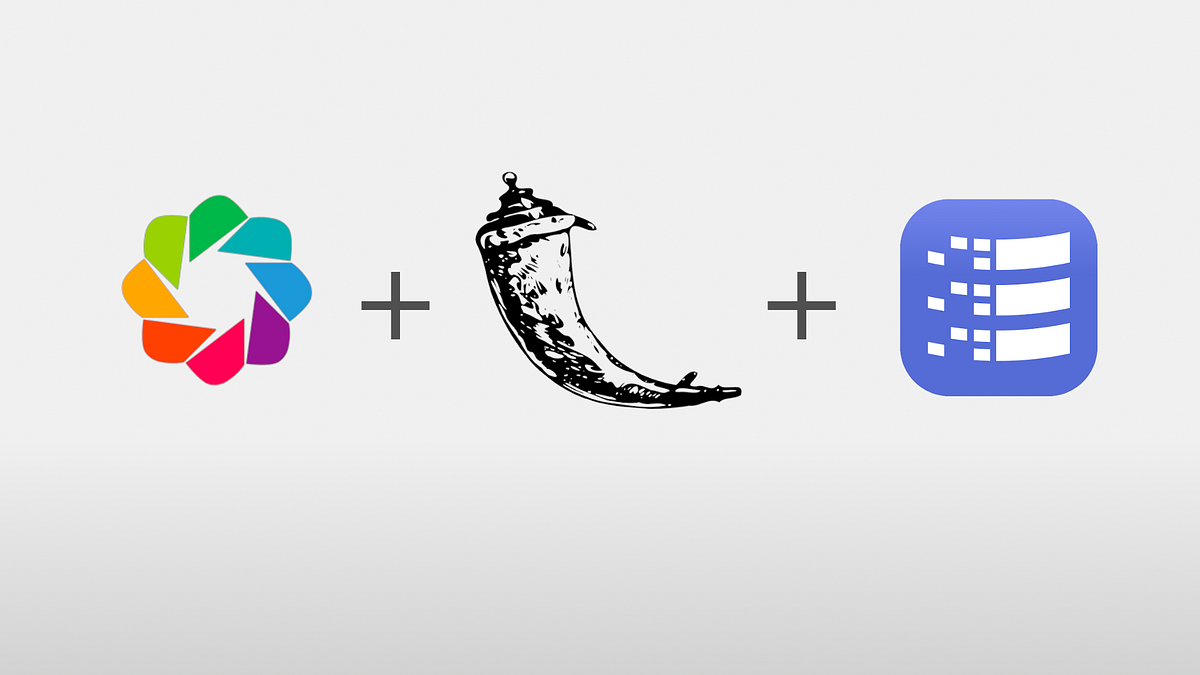1602788400

## How to Create an Animated Map of Bike Rentals in Chicago

This article assumes the reader already knows how to plot geographic data using the Bokeh library in Python. For an excellent and thorough explanation, please see this article written by Colin Patrick Reid.

Your data is processed and you’ve successfully adapted Colin’s code to create your own beautiful visualization in Bokeh, but you want to convey more information than is possible with a static image. You’re in the right place!

Initial Setup

We will start with this already processed data that combines bike rental data with historic daily average Chicago temperatures and the code below:

from bokeh.plotting import figure, show
from bokeh.tile_providers import get_provider, Vendors
import pandas as pd
from bokeh.models import ColumnDataSource, HoverTool
from bokeh.models import Label

path = 'C:\\Map'

df['circle_sizes'] = df['avg_trip_count'] / df['avg_trip_count'].max() * 40
temps = df['temp'].unique().tolist()

#only load in data for first temperature
df = df[df['temp']==df['temp'].max()]

source = ColumnDataSource(data=dict(
x=list(df['coords_x']),
y=list(df['coords_y']),
ridership=list(df['avg_trip_count']),
sizes=list(df['circle_sizes']),
stationname=list(df['station_name'])))

hover = HoverTool(tooltips=[
("station", "@stationname"),
("ridership","@ridership")

])

p = figure(x_range=(-9759380, -9749918), y_range=(5140778, 5150200),
x_axis_type="mercator", y_axis_type="mercator", tools=['pan',hover, 'wheel_zoom', 'save'])

p.circle(x = 'x',
y = 'y',
source=source,
size='sizes',
line_color="#FF0000",
fill_color="#FF0000",
fill_alpha=0.05
)

#Legend
rides = [50, 100, 200, 400]
circles = [x / df['avg_trip_count'].max() * 40 for x in rides]
x_coords = [-9752040] * 4
y_coords = [5148472, 5148124, 5147752, 5147133]
p.circle(x = x_coords, y = y_coords, size=circles, line_color="#FF0000", fill_color="#FF0000", fill_alpha=0.05)
p.add_layout(Label(x = -9752100, y=5148672, text='Rides Per Day'))

for x,y,text in zip(x_coords, y_coords, rides):
p.add_layout(Label(x = x+(500 if text!=50 else 595), y=y-200, text=str(text)))

loc =  (-9756040, 5148472)
mytext = Label(x=loc, y=loc, text='Temp: 80\N{DEGREE SIGN}F', text_font_size='25pt')
#end legend

show(p)


#data-visualization #python #data-science #bokeh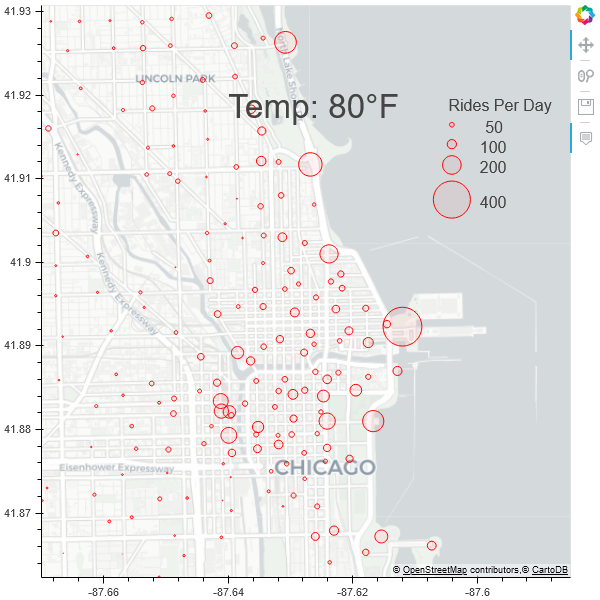1600890240

## Beginners Guide to Data Visualization with Bokeh

Bokeh is a data visualization library in Python. It provides highly interactive graphs and plots. What makes it different from other Python plotting libraries is that the output from Bokeh will be on the web page, meaning if we run the code in python editor the resulting plot will be in the browser. This gives the advantage of embedding the Bokeh plot on any website using Django or Flask.

Most of us are familiar with the iris dataset, it has morphological data of three different flower species namely Setosa, Virginica, and Versicolor. Let’s plot the above graph from scratch by learning the basics of Bokeh.

#exploratory-data-analysis #data-science #data-visualization #bokeh #data-analysis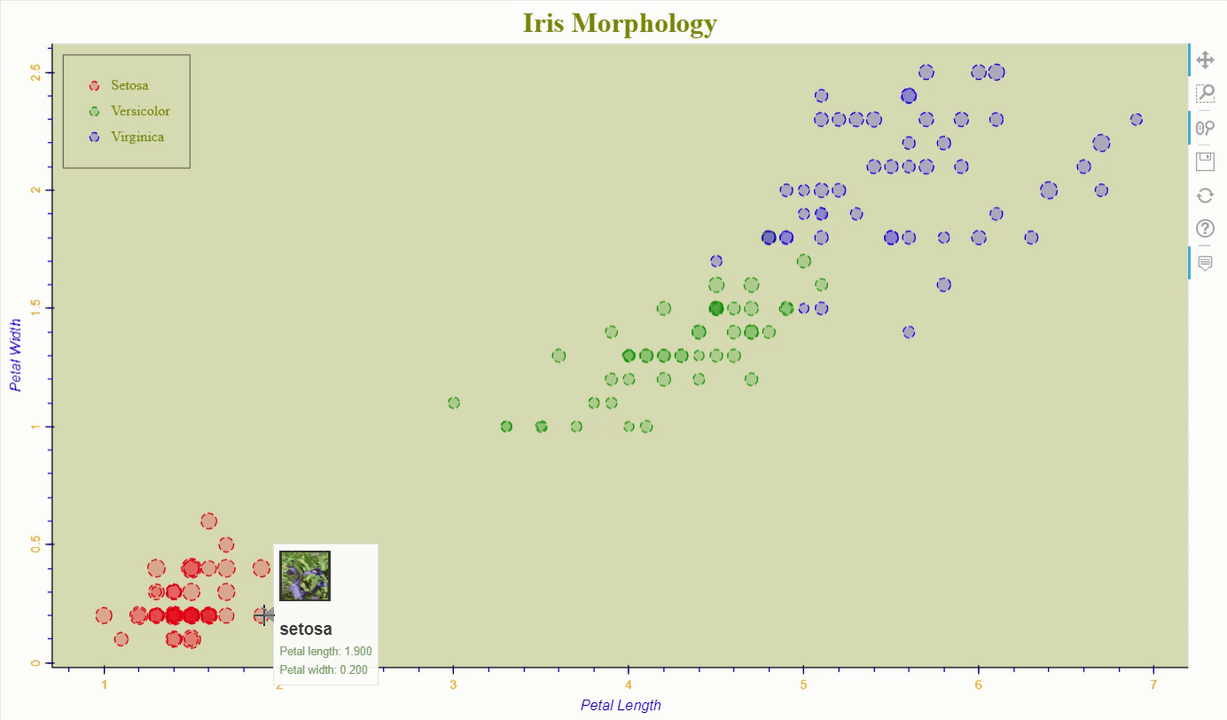1598904840

## Which library should I use for my dashboard?

When it comes to data visualization there are many possible tools Matplotlib, Plotly, Bokeh… Which one is fitting my short term goals, within a notebook, and is a good choice for longer-term, in production? What does production mean?

Now that you have a nice machine learning model, or you have completed some data mining or analysis, you need to present and promote this amazing work. You may initially reuse some notebooks to produce a few charts… but soon colleagues or clients are requesting access to the data or are asking for other views or parameters. What should you do? Which tools and libraries should you use? Is there a one fits all solution for all stages of my work?

Data-visualization has a very wide scope, ranging from presenting data with simple charts to be included in a report, to complex interactive dashboards. The first is reachable to anybody that knows about Excel whereas the later is more a software product that may require the full software development cycle and methodology.

In between these two extreme cases, data scientists face many choices that are not trivial. This post is providing some questions that will come along this process, and some tips and answers to these. The chosen starting point is Python within a Jupiter notebook, the target is a Web dashboard in production.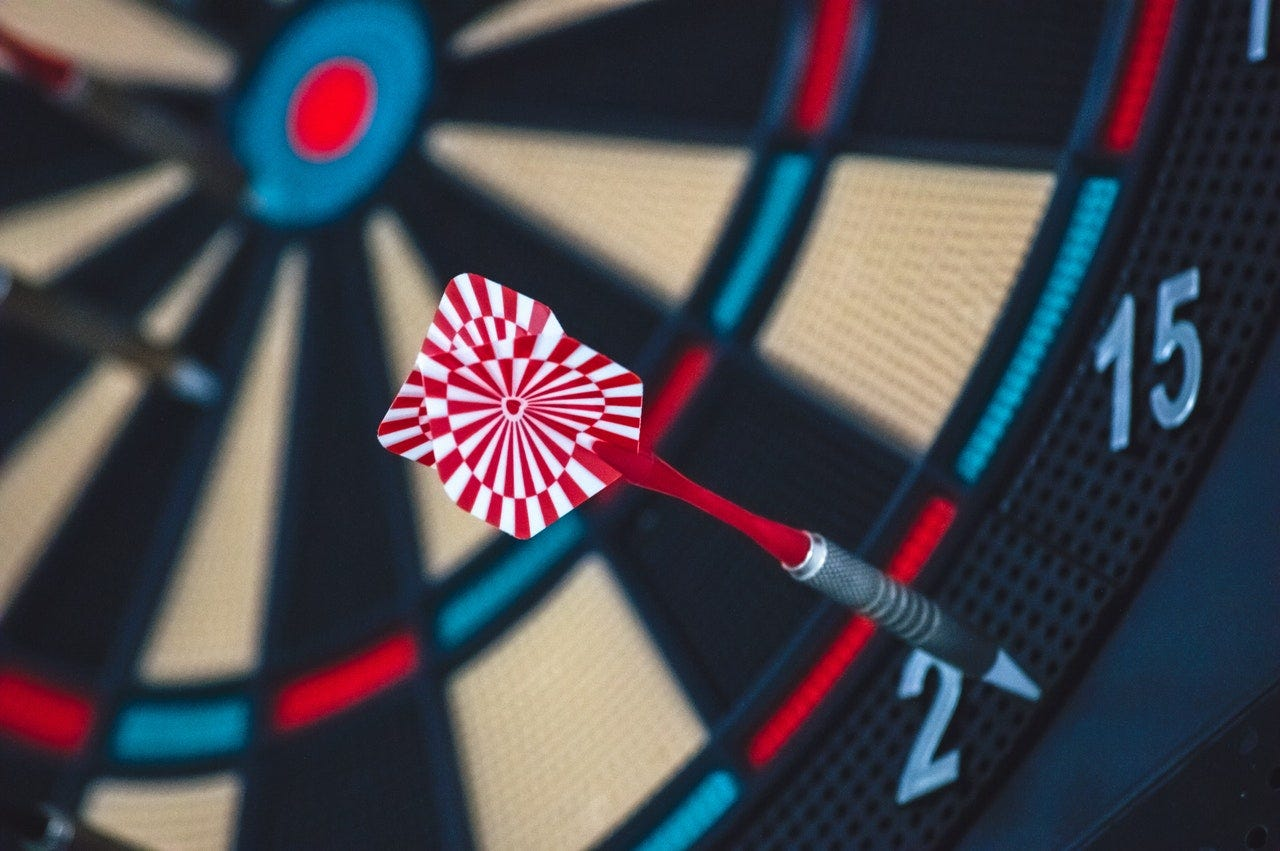#plotly #bokeh #data-visualization #matplotlib #data-science #big data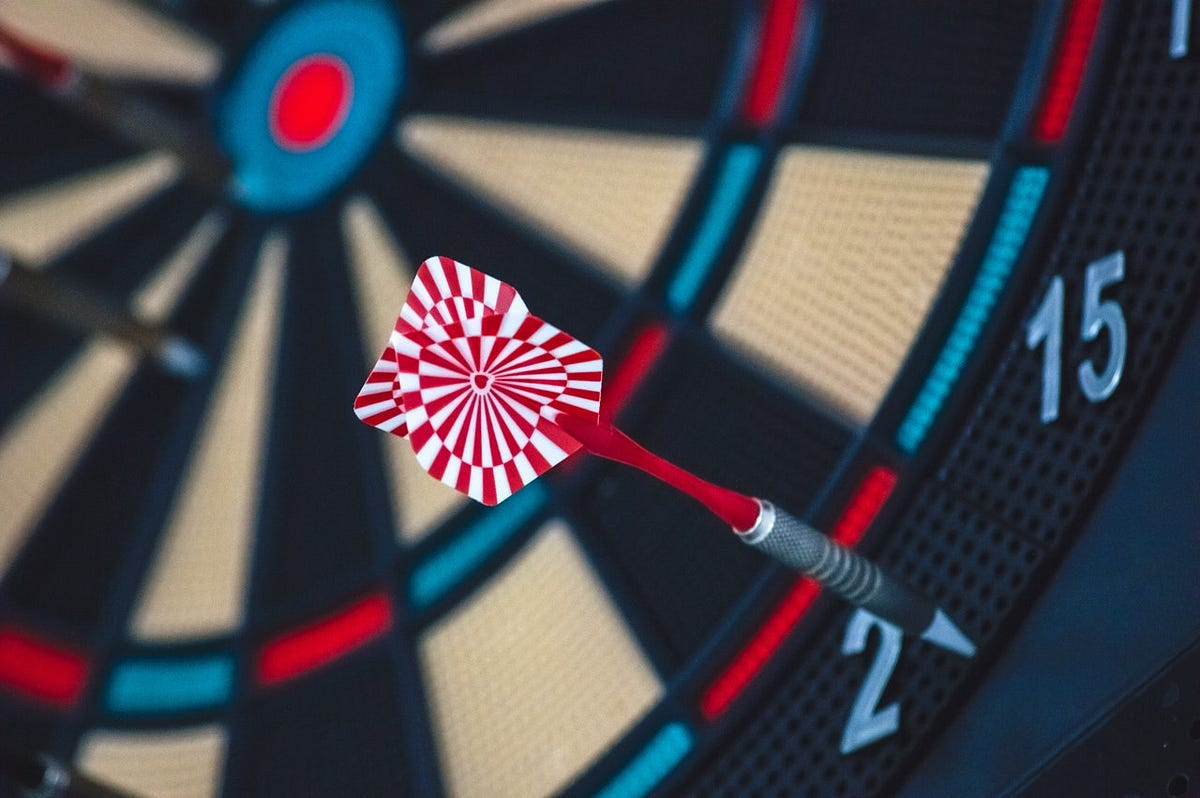1598587800

## Avalanche danger in France

Which mountain ranges are the most dangerous in France for hikers and alpinists? This was my main question, because I recently moved to Grenoble which is basically french hiking paradise.

Unfortunately, only regional data I have found about mountain accidents where yearly****_avalanche accidents from reports of _ANENA (organization for study of snow and avalanches in France) divided by communes (small administrative units in France). This was the second best thing to mountain accidents grouped by mountain ranges, which I was unable to find, so as in poker or tetris I played the hand I was dealt and searched for shapefile of communes in France(luckily, official sources of french gouvernment did not let me down). These were only two pieces for my visualization “puzzle” I needed to start coding in Jupyter Notebook to create interactive map of avalanche accidents in France in last 10 years.

### Don’t talk, just code

All files with source data and code in Jupyter notebook can be found in my GitHub repo Avalanche danger in France.

### 1) Installation

Assuming you have already standard Python libraries like Pandas and Numpy installed, for handling geospatial data and shapefiles I needed to add GeoPandas and Bokeh.

In my case (Windows with Anaconda) magical words for my command line were; _conda install geopandas and conda install bokeh, _as it is advised in documentation of GeoPandas and Bokeh libraries.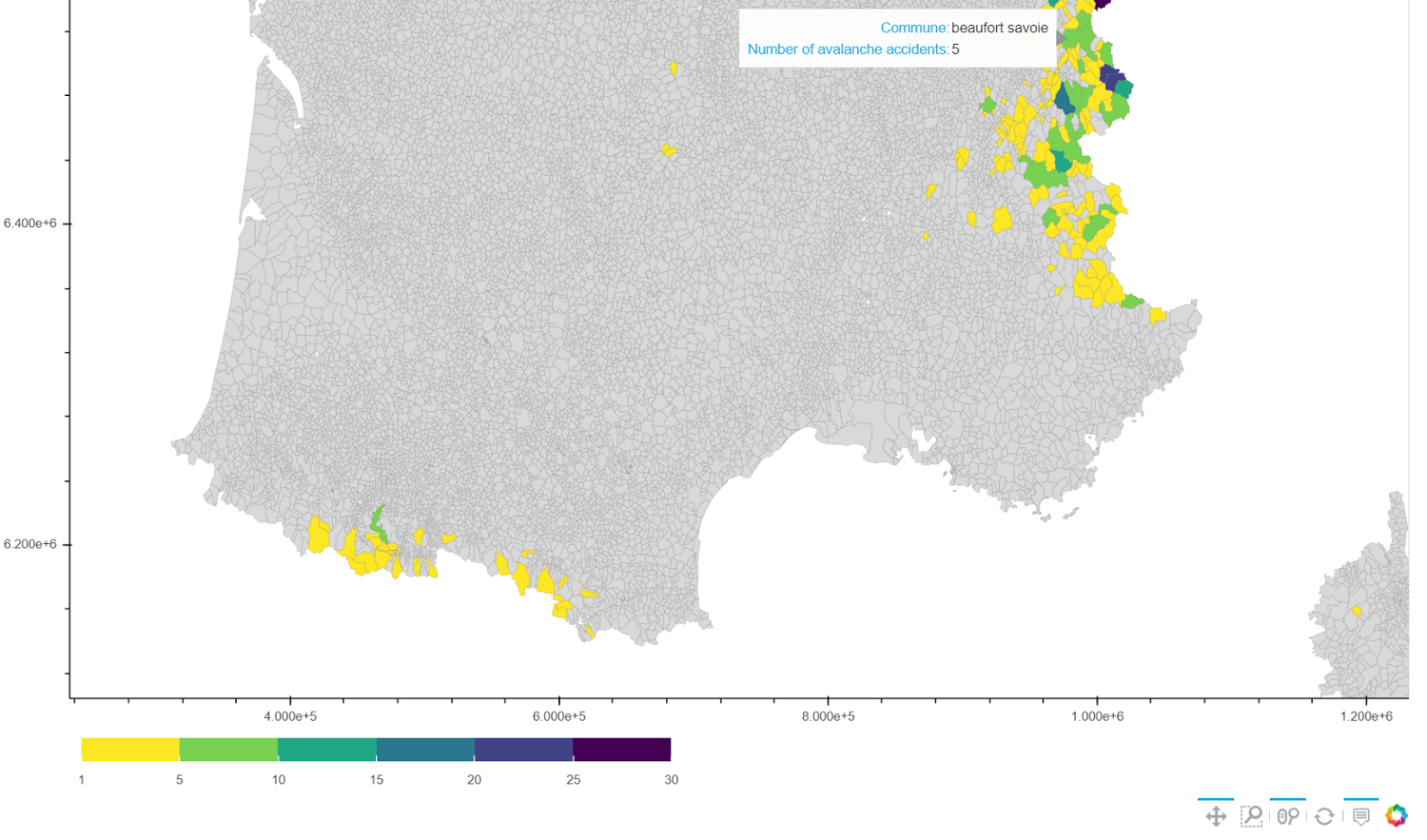Preview of final visualization depicting in colors commune with 1 - 30 avalanches in last 10 years in France and communes with no avalanches in grey

#jupyter-notebook #python #bokeh #france #avalanche1598341020

## Data Visualization Using Pandas Bokeh

### Create stunning visualizations for Pandas DataFrames

Exploratory data analysis is the foundation for understanding and building effective ML models. Data visualization is a key part of EDA, and there are many tools available for this. Bokeh is an interactive visualization library. It provides intuitive and versatile graphics. Bokeh can help to quickly and easily make interactive plots and dashboards. Pandas Bokeh provides a Bokeh plotting backend for Pandas.

Integrating Pandas Bokeh with your Python code is very simple. You only need to install and import the pandas-bokeh library, and then you can use it like any other visual tool. You should import Pandas-Bokeh library after importing Pandas. Use the following command to download and import pandas-bokeh library:

#Load the pandas_bokeh library

!pip install pandas_bokeh

import pandas as pd
import pandas_bokeh


You can set the plotting output as HTML or Notebook. To set the output to notebook use the command, pandas_bokeh.output_notebook(). This will embed the plot in the notebook cell. To display the output as a HTML file use the command, pandas_bokeh.output_file(filename).

You can easily plot Pandas DataFrames using the command, df.plot_bokeh(). Pandas Bokeh offers a wide variety of plotting options such as line, scatter, bar, histogram, area, mapplot, step, point, and pie. All the plots are interactive, pannable, and zoomable. Here are some examples with the code of popular visualizations, plotted using pandas_bokeh that are commonly used in data analysis.

Bar Plot

#Vertical barchart
carhpbot.plot_bokeh(
kind="bar",
figsize =(1000,800),
x="name",
xlabel="Car Models",
title="Bottom 10 Car Features",
alpha=0.6,
legend = "top_right",
show_figure=True)

#Stacked vertical bar
carhpbot.plot_bokeh.bar(
figsize =(1000,800),
x="name",
stacked=True,
xlabel="Car Models",
title="Bottom 10 Car Features",
alpha=0.6,
legend = "top_right",
show_figure=True)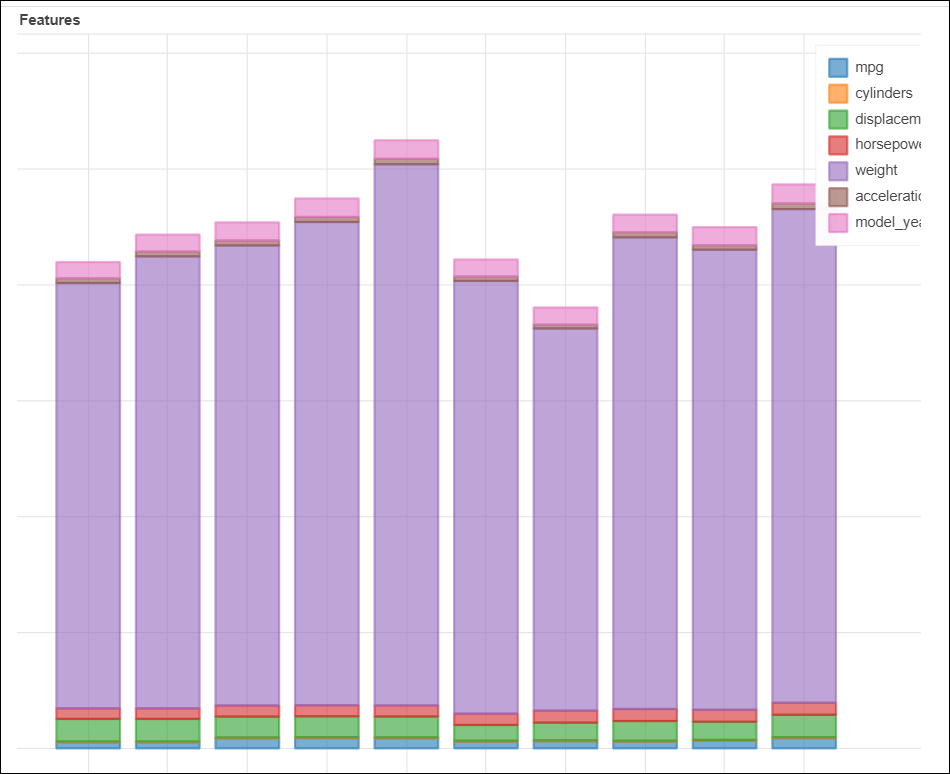#data-visualization #exploratory-data-analysis #data-analysis #bokeh #pandas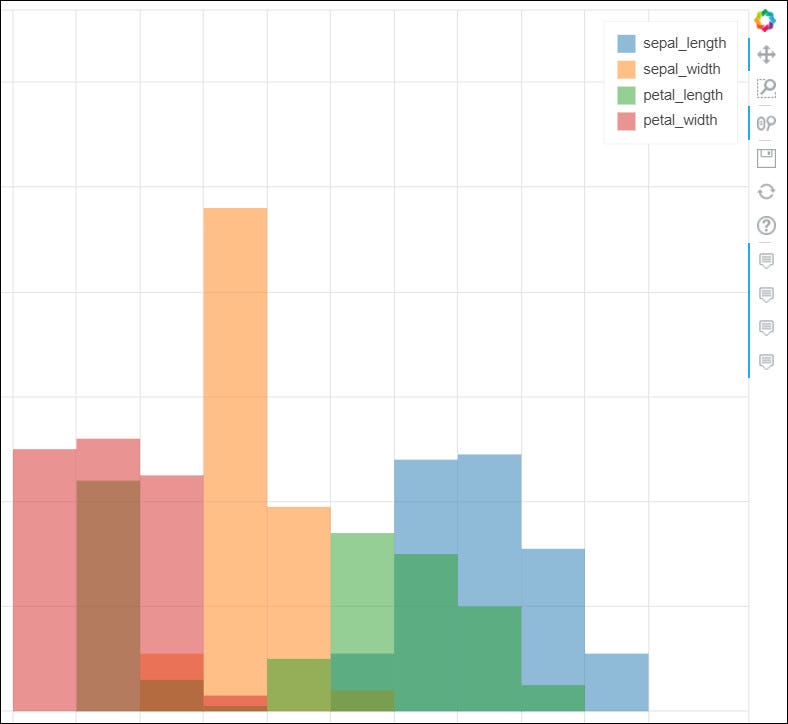1597378500

## Open Source Python Library For Interactive Visualizations

Dashboards are collections of bars, charts, and graphs that help us visualize different attributes of a dataset. A dashboard works as a graphical user interface which helps us identify the key performance indicators relevant to the dataset or the particular business model. Python provides different open-source libraries that can help you create your own dashboard with your dataset. Today we will be talking about Bokeh which is an open-source python library for interactive visualizations for the modern web browsers.

Bokeh provides elegant, concise construction of versatile graphics, and affords high-performance interactivity over large or streaming datasets. It can be used for different purposes like creating interactive plots, dashboards, and even data-driven applications.

1. Creating Bokeh Visualization and Analyzing it.
2. Creating a Sales Dashboard using Bokeh

#### Implementation of Bokeh:

Like any other library, we need to install Bokeh for exploring it by pip install bokeh

1. Importing required libraries

We will import pandas for loading the dataset and will import different functions of bokeh as and when required.

import pandas as pd

from bokeh.plotting import figure, output_file, show

We will create a sales dashboard for which we need sales data of a company, here I will use a dataset which contains Sales of a company and different attributes on which it depends.

df = pd.read_csv(‘Advertising.csv’)

df#developers corner #bokeh #data analysis #data analytics #sales analytics tools #data analysis1597059118

## Hands-On Tutorial on Bokeh - Open Source Python Library For Interactive Visualizations

Dashboards are collections of bars, charts, and graphs that help us visualize different attributes of a dataset. A dashboard works as a graphical user interface which helps us identify the key performance indicators relevant to the dataset or the particular business model. Python provides different open-source libraries that can help you create your own dashboard with your dataset. Today we will be talking about Bokeh which is an open-source python library for interactive visualizations for the modern web browsers.

#datavisualization #data #dashboard #learndatascience #bokeh #analytics1593263820

In this series of articles, I’m looking at the characteristics of different Python plotting libraries by making the same multi-bar plot in each one. This time I’m focusing on Bokeh (pronounced “BOE-kay”).

Plotting in Bokeh is a little more complicated than in some of the other plotting libraries, but there’s a payoff for the extra effort. Bokeh is designed both to allow you to create your own interactive plots on the web and to give you detailed control over how the interactivity works. I’ll show this by adding a tooltip to the multi-bar plot I’ve been using in this series. It plots data from UK election results between 1966 and 2020.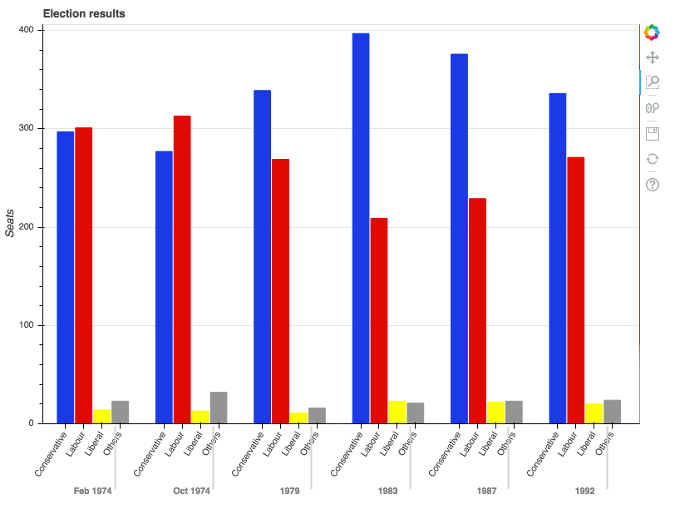## Making the multi-bar plot

Before we go further, note that you may need to tune your Python environment to get this code to run, including the following.

• Running a recent version of Python (instructions for LinuxMac, and Windows)
• Verify you’re running a version of Python that works with these libraries

The data is available online and can be imported using pandas:

import pandas as pd


To make the multi-bar plot, you need to massage your data a little.

The original data looks like this:

>> print(long)
year    party seats
0   1966 Conservative  253
1   1970 Conservative  330
2 Feb 1974 Conservative  297
3 Oct 1974 Conservative  277
4   1979 Conservative  339
..   ...     ...  ...
103  2005    Others  30
104  2010    Others  29
105  2015    Others  80
106  2017    Others  59
107  2019    Others  72

[60 rows x 3 columns]


You can think of the data as a series of **seats** values for each possible **(year, party)** combination. That’s exactly how Bokeh thinks of it. You need to make a list of **(year, party)** tuples:

# Get a tuple for each possible (year, party) combination
x = [(str(r['year']), r['party']) for r in df.iterrows()]

# This comes out as [('1922', 'Conservative'), ('1923', 'Conservative'), ... ('2019', 'Others')]


These will be the x-values. The y-values are simply the seats:

y = df['seats']


Now you have data that looks something like this:

x               y
('1966', 'Conservative')    253
('1970', 'Conservative')    330
('Feb 1974', 'Conservative')  297
('Oct 1974', 'Conservative')  277
('1979', 'Conservative')    339
...   ...         ...
('2005', 'Others')       30
('2010', 'Others')       29
('2015', 'Others')       80
('2017', 'Others')       59
('2019', 'Others')       72


Bokeh needs you to wrap your data in some objects it provides, so it can give you the interactive functionality. Wrap your x and y data structures in a **ColumnDataSource** object:

   from bokeh.models import ColumnDataSource

source = ColumnDataSource(data={'x': x, 'y': y})


Then construct a **Figure** object and pass in your x-data wrapped in a **FactorRange** object:

   from bokeh.plotting import figure
from bokeh.models import FactorRange

p = figure(x_range=FactorRange(*x), width=2000, title="Election results")


You need to get Bokeh to create a colormap—this is a special **DataSpec** dictionary it produces from a color mapping you give it. In this case, the colormap is a simple mapping between the party name and a hex value:

   from bokeh.transform import factor_cmap

cmap = {
'Conservative': '#0343df',
'Labour': '#e50000',
'Liberal': '#ffff14',
'Others': '#929591',
}
fill_color = factor_cmap('x', palette=list(cmap.values()), factors=list(cmap.keys()), start=1, end=2)


Now you can create the bar chart:

  p.vbar(x='x', top='y', width=0.9, source=source, fill_color=fill_color, line_color=fill_color)


Visual representations of data on Bokeh charts are referred to as glyphs, so you’ve created a set of bar glyphs.

Tweak the details of the graph to get it looking how you want:

  p.y_range.start = 0
p.yaxis.axis_label = 'Seats'
p.xaxis.major_label_orientation = 1
p.xgrid.grid_line_color = None


And finally, tell Bokeh you’d like to see your plot now:

  from bokeh.io import show

show(p)


This writes the plot to an HTML file and opens it in the default web browser. Here’s the result: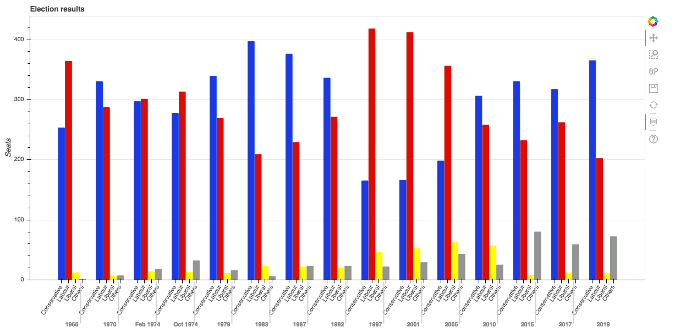This already has some interactive features, such as a box zoom: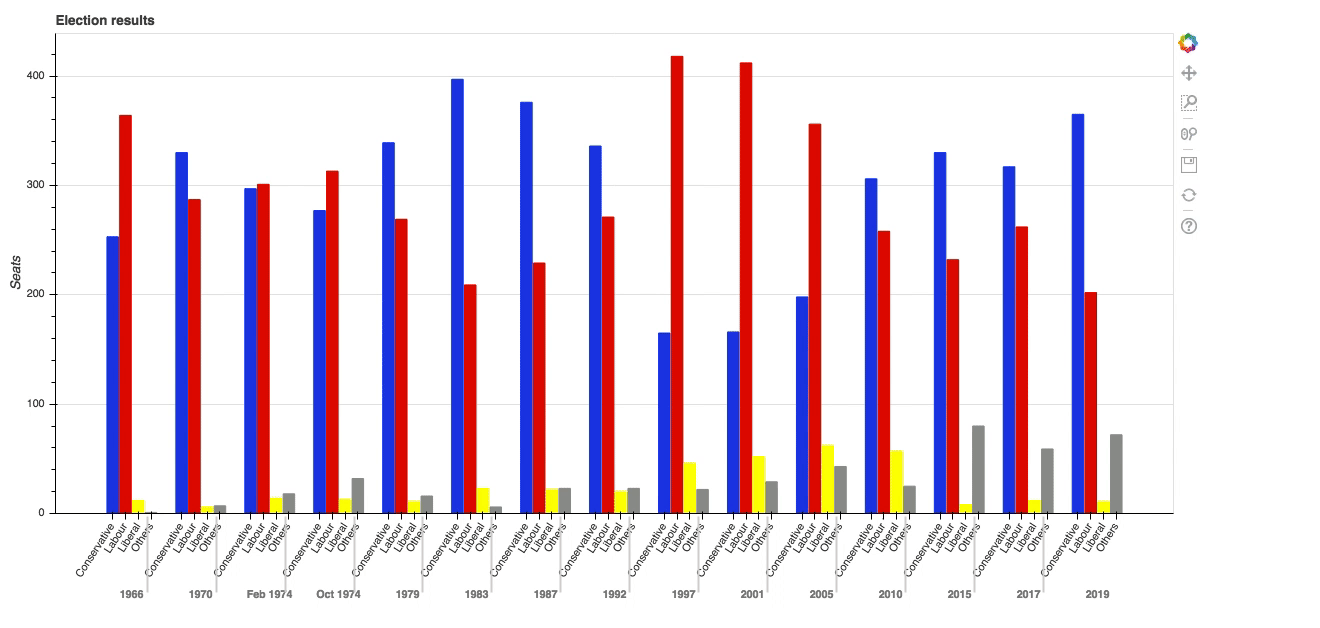But the great thing about Bokeh is how you can add your own interactivity. Explore that in the next section by adding tooltips to the bars.

#python #bokeh1591926480

## Creating a map of house sales

In this tutorial, I will guide you step by step to create a map displaying houses sales using Bokeh, with a colour mapping indicating sale price. I wanted the viewer to be able to distinguish at a glance which neighbourhoods are most expensive to live in .

#bokeh #python